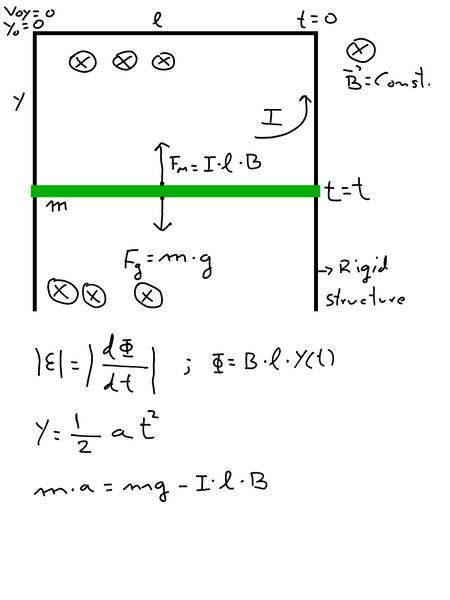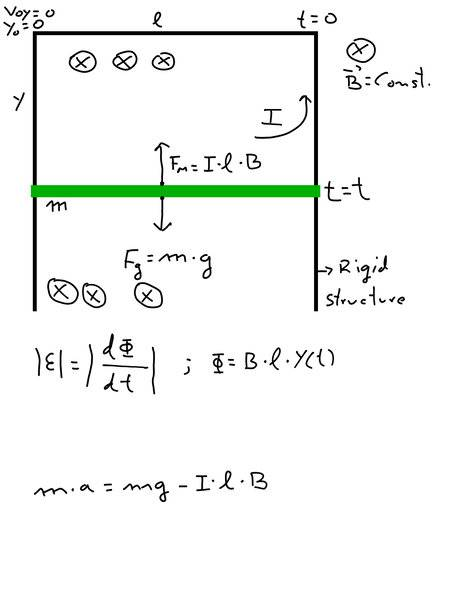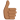# Metal Stick that falls by gravity inside a magnetic field

## Homework Statement

I am trying to solve a problem that I have invented.

It consists of a metal stick of mass m, which falls by the action of gravity (g=constant) within a perpendicular constant magnetic field B. The direction of magnetic fiel is showed in the image.
The stick makes electrical contact with a rigid metal structure. (view image).This generates an intensity I(t) . And this intensity generates a force on the bar that according to Lenz's law is upwards (this is the big problem)
Initial positions and velocity are showed in the image. For easiest calculations they are Vo=0 and Yo=0.

## Homework Equations

$$|\varepsilon|=\left| \frac {d\Phi} {dt} \right|$$$$\Phi=B·l·y(t)$$$$y(t)=1/2·a(t)·t²$$

## The Attempt at a Solution

I use the Second Newton's Law: $$m·a=m·g-I(t)·l·B$$
for calculate the acceleration.
But I don't know if I need to know the value of the circuit resistance, of if I can solve it with the square loop induction coefficient L (Henrios). I need to calculate I(t) and I don't know how I can do it.

#### Attachments

Delta2
Homework Helper
Gold Member
if we want to treat fully this problem, (including self induction, capacitance between the conductors of the rigid structure and the metal stick, and electromagnetic radiation) , we ll have to write a mini master thesis.
So we have to make some simplifying but realistic assumptions:

1) both self induction and radiation can be considered to be negligible (unless the metal stick is allowed to gain really big speeds which will lead to large values for the current and voltage produced). Also negligible is the capacitance of the rigid structure.

2) Due to 1) we need to have some ohmic resistance in the square loop , otherwise the current will be infinite. Another simplifying assumption here would be that the resistance of the vertical conductors is zero, so we consider only the resistance of the metal stick and the horizontal conductor of length l that is parallel to it.

Your equation you got from applying newton second law is correct. Have in mind that the acceleration a there relates to the velocity of the metal stick as ##a=\frac{dv}{dt}##.

Your equation for y(t) is correct only if acceleration is constant, otherwise it isn't correct. In any case, it would be better to use the ##E=Bv(t)l## formula for the emf produced, where ##v=v(t)=\frac{dy}{dt}## is the instantaneous velocity of the metal stick.

What is another equation you can make by applying Kirchoff's Voltage Law for the square loop (give resistance ##R_s## to the stick and ##R_l## to its parallel piece of conductor)?

So at the end you ll have two equations (differential equations) with two unknowns, the velocity of the stick ##v(t##) and the current ##I(t)##.

Last edited:
•alejandromeira
Your equation for y(t) is correct only if acceleration is constant, otherwise it isn't correct. In any case, it would be better to use the E=Bv(t)lE=Bv(t)lE=Bv(t)l formula for the emf produced, where v=v(t)=dydtv=v(t)=dydtv=v(t)=\frac{dy}{dt} is the instantaneous velocity of the metal stick.
What is another equation you can make by applying Kirchoff's Voltage Law for the square loop (give resistance RsRsR_s to the stick and RlRlR_l to its parallel piece of conductor)?
I suposse Ohm Law ##\varepsilon (t)=I(t)·(R_s + R_l)##. Rtotal is a constant, is simply R, I think.

Then I will re-upload the image by removing the wrong equation.Ok. Thank you. I will try to solve it with your suggestions. This can take me some time.

#### Attachments

Delta2
Homework Helper
Gold Member
Yes your equation is correct again.

I made a mistake in what I said in 1) in my first post, there will be a steady state in this problem which will prevent the stick from reaching too high velocities (unless it is starting with high initial velocity), if you are interested only for the steady state, you can find the current I for the steady state by setting a=0 in your 1st equation. Then from 2nd equation (ohms law as you said) you can find the velocity v of the steady state. (have in mind that E=Bvl)

If you are interested for the transient state, you got to solve the system of the two differential equations.

•alejandromeira
Ok. I will follow your suggestions and start with the simplest case you say with the resistance R. Once this is done I will try to go to the other cases. Give me some time until tomorrow.

•Delta2
I obtained the first differential equation in the simplest case of R and Ohm Law. Ok I need some time for solve it. Also tomorrow embarrassing me a familiar convocation, and I will not be able to work on the problem. Ok, when I have the result I will post it with the complete deduction

Delta2
Homework Helper
Gold Member
Ok fine, just to let you know, if you want also to include the increasing resistance (as y(t) will be increasing) of the vertical conductors, then the differential equation is gonna become 2nd order ,. and (this is the worst part) non-linear.

And if at next step you will also include the self induction of the increasing square loop, they gonna be two very ugly, non linear, coupled differential equations of 2nd order.

Finally it was easier than I thought for Resistance only. $$\Phi=B·l·y$$ and $$\frac {d\Phi}{dt}=B·l·v=I·R$$ and then $$I=\frac {B·l} {R}·v$$Second Newton's Law:$$m·a=m·g-I·l·B$$$$m·\frac {dv}{dt}=m·g-\frac {B·l} {R}·v·l·B$$$$m·\frac {dv}{dt}=m·g-\frac {B^2·l^2} {R}·v$$ and then: $$\frac {dv}{dt}=g-\frac {B^2·l^2} {m·R}·v$$ This is a differencial lineal ordinary equation in the first order. Type: $$f'=C·f+D$$where: ##C=-\frac {B²·l²}{m·R}##, and ##D=g##
I'm not specialist in differencial equiations (and this seems too easy) and then I use WolframAlpha online to solve it. Solution is:$$f(t)=K·e^{C·t}-\frac D C$$And then: $$v(t)=K·e^{-\frac{B²·l²}{m·R}·t}+\frac{g·m·R}{B²·l²}$$Then our initial conditions is Vo=0 at t=0. We can compute the constant K.$$K=-\frac{g·m·R}{B²·l²}$$Finally we organize the expression as (solution): $$v(t)=\frac{g·m·R}{B²·l²}·\left( 1-e^{-\frac{B²·l²}{m·R}·t}\right)$$
This function shows a exponential growth for time small, but when t increases the exponential term vanishes and velocity is a constant ##v(cte)=\frac{g·m·R}{B²·l²}##
Ok, this is what I was looking for, and it's the problem that I had imagined. I was curious to know how the stick falls.
And if at next step you will also include the self induction of the increasing square loop, they gonna be two very ugly, non linear, coupled differential equations of 2nd order.
Of course. I want to learn how to solve this. There are one equation for R and another for L, or is another term in the ##\varepsilon## expression.
I'm sorry I don't know how begin.
Thanks

•Delta2
Delta2
Homework Helper
Gold Member
what case do you want to do now? The one where we take into account the increasing (as y increases) resistance from the vertical conductors, or the self inductance of the square loop? or both?

•alejandromeira
I prefers the self inductance of the sqare loop : -)
Maybe it's: ##\varepsilon=I·R-L·\frac{dI}{dt}## ??
How I can begin??Delta2
Homework Helper
Gold Member
I prefers the self inductance of the sqare loop : -)
Maybe it's: ##\varepsilon=I·R-L·\frac{dI}{dt}## ??
How I can begin??Almost. The big problem here is that L is not constant but its a function of time because as time passes the loop increases in area.

First we should find an expression for the total flux through the loop, and then use ##\frac{d\Phi}{dt}=IR##

The crucial change regarding the total flux is that now it will be ##\Phi=\Phi_B+\Phi_{B_I}## where ##\Phi_B## the flux due to the homogeneous constant magnetic field , and ##\Phi_{B_I}## the flux due to the magnetic field from the current I.

We already know ##\Phi_B## so we ll focus how to find ##\Phi_{B_I}##. A good approximation for ##B_I(t)=kI(t)## where k a constant which we ll find later. So how would you proceed for finding ##\Phi_{B_I}## given that ##B_I##. Once you find, what can you say about the derivative ##\frac{d\Phi_{B_I}}{dt}##?

Ok, yes I know L is not a constant. I've made this: $$\Phi_{B_{I}}=k·I(t)·l·y$$ We know ##L=\frac {\Phi_{B_{I}}}{I(t)}##, then: ##L=k·l·y##
Now I calculate: $$\frac {d\Phi_{B_{I}}}{dt}=k·l·\frac {d}{dt}\left\{I(t)·y(t)\right\}$$ and
$$\frac {d\Phi_{B_{I}}}{dt}=k·l·\frac {dI(t)}{dt}·y+k·l·I(t)·v$$
And ##L=k·l·y##
then finally I've obtained:
$$\frac {d\Phi_{B_{I}}}{dt}=L\frac {dI(t)}{dt}+k·l·I(t)·v$$
Ok I don't know if I go in the right way.

Another thing, ##\Phi_{B_{I}}## is oposite to ##\Phi_{B}## I suposse, then ##\Phi_{total}## must be in substraction not in adition. (??)

Ok today is finish, in my country now it's too late.

Delta2
Homework Helper
Gold Member
Ok, yes I know L is not a constant. I've made this: $$\Phi_{B_{I}}=k·I(t)·l·y$$ We know ##L=\frac {\Phi_{B_{I}}}{I(t)}##, then: ##L=k·l·y##
Now I calculate: $$\frac {d\Phi_{B_{I}}}{dt}=k·l·\frac {d}{dt}\left\{I(t)·y(t)\right\}$$ and
$$\frac {d\Phi_{B_{I}}}{dt}=k·l·\frac {dI(t)}{dt}·y+k·l·I(t)·v$$
And ##L=k·l·y##
then finally I've obtained:
$$\frac {d\Phi_{B_{I}}}{dt}=L\frac {dI(t)}{dt}+k·l·I(t)·v$$
Ok I don't know if I go in the right way.
Correct but I don't consider it a good idea to put ##L=kly##, because ##y=y(t)## and ##v=dy/dt##, in the differential equation for ##v## we gonna get after, we may be fooled and think that L is a constant but it depends on ##y## !. In fact it would be better to express the differential equation with respect to ##y,dy/dt,d^2y/dt^2##. I got a feeling the differential equation for y(t) (and for current I(t)) this time is not gonna be linear.

Another thing, ##\Phi_{B_{I}}## is oposite to ##\Phi_{B}## I suposse, then ##\Phi_{total}## must be in substraction not in adition. (??)
Correct again, Thanks for the correction !.

Last edited:
•alejandromeira
Ah! Ok, then now perhaps I understand the problem: using the previous results we have a differential equation for epsilon = I · R, and the other diferential equation is the Second Newton's Law. Then we have a system of two differential equations and one is of second order. Ok solve this system really is a course in differential equations!!
Using the above results: $$\frac{d\Phi}{dt}=\frac{d\Phi_{B}}{dt}-\frac{d\Phi_{B_{I}}}{dt}=I·R$$$$B·l·v-k·l·\frac{dI(t)}{dt}·y-k·l·I(t)·v=I(t)·R$$
Second Newton's Law: $$m·\frac{dv}{dt}=m·g-I(t)·l·B$$
I translate these equations to the notation: y(t), y'(t), y''(t), I(t), I'(t)
$$B·l·y'(t)-k·l·I'(t)·y(t)-k·l·I(t)·y'(t)=I(t)·R$$$$m·y''(t)=m·g-I(t)·l·B$$
that's ok???
Another thing, the constant k, I suposse will be related with ##\mu_0## because the definition of ##B_I## ???

Edit: Before to solve this system I think if some terms are negligible, perhaps due the small value of ##\mu_0## the terms in k, (also we have only one loop). But if the terms in k vanishing then we are in the previous problem.

Last edited:
•Delta2
Delta2
Homework Helper
Gold Member
The way I calculated the constant k is to consider the magnetic field ##B_I## homogeneous inside the square loop and then use Ampere's law with an Amperian loop that has dimensions ##d\cdot y## where d is the diameter of the conductors. So it will be ##Bd=\mu_0 I(t)\Rightarrow B=\frac{\mu_0}{d}I(t)##. So yes ##k=\frac{\mu_0}{d}## will be small, but it is multiplied by ##l## and still it is interesting to see how the differential equations get more complicated because of k.

There is also another way to do this, by getting the## L## , where ##\Phi_{B_I}=LI## directly from using the Neumann Formula https://en.wikipedia.org/wiki/Inductance#Self-inductance_of_a_wire_loop

and the result of that integration is mentioned in this board ("rectangle made of round wire" is our case) https://en.wikipedia.org/wiki/Inductance#Self-inductance_of_thin_wire_shapes,

you can see that the L there is pretty complicated , it has lots of terms.

So better use our approach where I essentially calculate the ##B_I## as that B of a solenoid with just one turn and length equal to the diameter of the conductors.

Last edited:
Ok, this is enough for me, because I have understood the problem.
If we want to be more precise in the value of Bind, that would be to solve another different problem.
Also if, Bind, is moore complicated the system of equatios is more hard too. Perhaps only one can solve them with a numerical approximation.

Ok, with some time (and a computer) I will try to find the equation system solution.

I have learned more than I expected, for my part the problem is solved.
Ok thanks a lot!!!•Delta2
Delta2
Homework Helper
Gold Member
If we want to be more precise in the value of Bind, that would be to solve another different problem.
Also if, Bind, is moore complicated the system of equatios is more hard too. Perhaps only one can solve them with a numerical approximation.
Totally agree with you here.
Ok thanks a lot!!!Was a pleasure meeting you in these forums and cooperating with you.